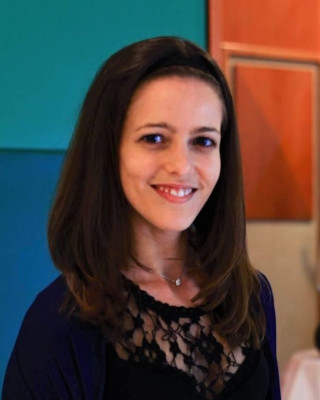The research of the Algebra and Number Theory Group at the Institute of Mathematics and Scientific Computing at the University of Graz (Austria) spans topics from (commutative and noncommutative) ring theory and module theory. We are particularly interested in the arithmetic of rings and monoids, the interaction between their arithmetic and their algebraic structure, their multiplicative ideal theory, and their factorization theory. The monoids we mainly have in mind stem from ring and module theory. For instance, we study the structure of monoids of modules in order to understand their direct-sum decompositions.

Studying factorizations of elements into irreducibles, transfer techniques allow to shift arithmetical questions in rings to problems in additive combinatorics. Thus, topics in additive combinatorics, including zero-sum problems, addition theorems, and polynomial methods, also count among our research interests.

We regularly collaborate with international colleagues from (amongst others) Canada, China, France, Italy, and the United States, and we participate in the FWF funded Doctoral Program Discrete Mathematics and the Doctoral Academy Graz.

# Researchers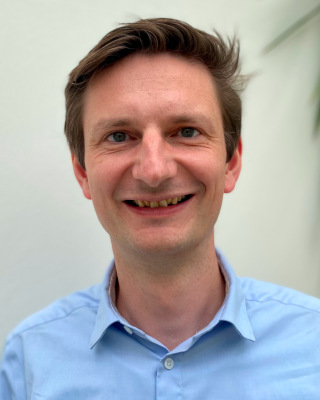Daniel Smertnig, Univ.-Prof. (Head) Noncommutative rings, factorization theory, quaternion algebras, noncommutative rational series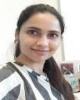Aqsa Bashir, MPhil (PhD student) Multiplicative ideal theory, factorization theory, zero-sum theory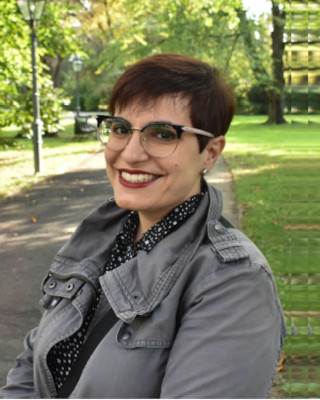Laura Cossu, PhD Commutative rings, matrix theory, factorization theory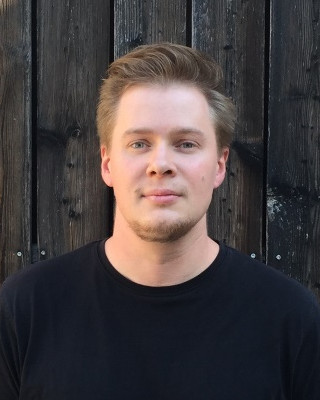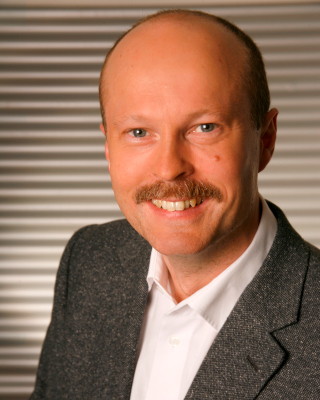Alfred Geroldinger, Ao. Univ.-Prof. Multiplicative ideal theory, factorization theory, additive combinatorics, zero-sum theoryFranz Halter-Koch, Univ.-Prof. (retired) Algebraic number theory, multiplicative ideal theory, factorization theory, quadratic irrationalsFlorian Kainrath, Ass.-Prof.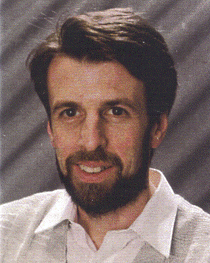Günter Lettl, Ao. Univ-Prof. (retired)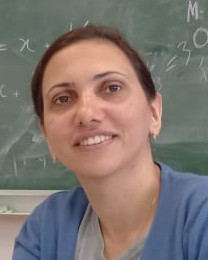Zahra Nazemian, PhD Module theory, Noncommutative algebra and ring theory, Homological algebra and its application in ring theory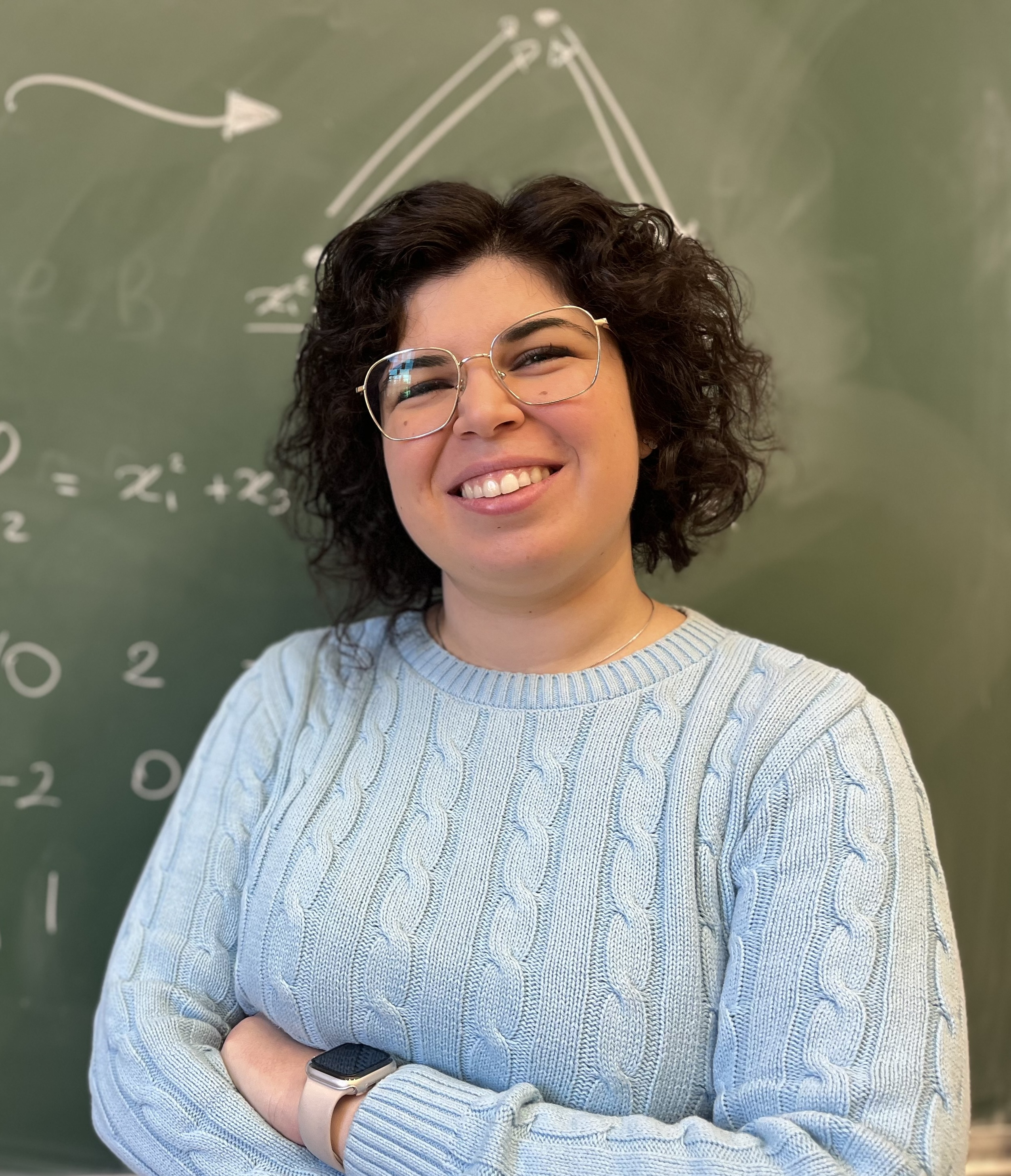Mara Pompili, Dott. Mag. (PhD student)Balint Rago, MSc (PhD student)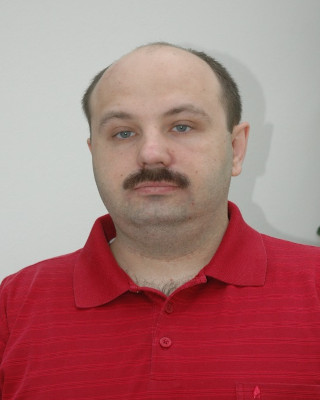Andreas Reinhart, Priv.-Doz. Commutative rings, multiplicative ideal theory, factorization theory